## Home School Life Journal From Preschool to High School

Home School Life Journal ........... Ceramics by Katie Bergenholtz
"Let us strive to make each moment beautiful."
Saint Francis DeSales

## Our Homeschool From Preschool to High School

### Place Value with Decimals

1. Review place value in general. Read How Much is a Million? by David Schwartz. Practice reading and writing large numbers in numeral and written form. David Schwartz tells us that it takes one gallon of water to keep a one-inch goldfish alive. How many gallons of water will it take to keep 10 goldfish alive? 100 goldfish? 10, 000 goldfish? One million goldfish? There are 7 1/2 gallons of water in a cubic foot of water. How many cubic feet of water are necessary to keep 10 goldfish alive? 100 goldfish? 10, 000 goldfish? One million goldfish?
2. Begin demonstrating decimals on a trading board.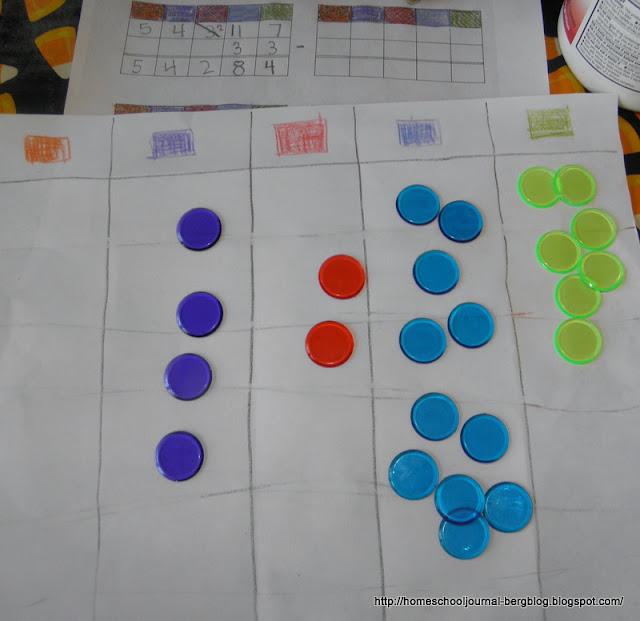If you used one of these to introduce addition and subtraction, now you can just shift your child's thinking by telling him the one cup on the trading board is now one, instead of ten, then one bean is 1/10. I told him to think of it as if the cup represented a class at co-op which had 10 students in it. The class would be one, and each student would be 1/10 of the class. If we decided that the bowl represented one (instead of 100 as it had in the past), then we could look at it as one co-op school, in which each class was 1/10 of the co-op (assuming that the co-op had 10 classes in it) and each student was 1/100 of the co-op students. After this introduction, we practiced ways of writing fractions whose denominators are powers of ten. We wrote them as fractions and we wrote them as decimals.
3. Next, practice the same concept using different materials.Assign fractional values using plastic colored chips on the counting/trading board. Together create decimal problems for addition and subtraction using the counting/trading board by rolling a die for each column. Since he already knows how to create addition and subtraction problems in this way, the only difficulty he might encounter is where to place the decimal.
4. Next get out a piece of 10 x 10 graph paper to make a matrix. You can use Math-U- See blocks, Unifix cube sticks, bean sticks or whatever you have as a base-10 manipulative, since we are working with decimals, which are all working with divisions of ten. Use whatever you had used to represent 10 and call it one. Using a series of questions, you can guide your student to see the relationships.5. Have the student read decimal fractions through ten thousandths and decimal fractions with whole numbers. Have your student write the following rules in his math journal: 1.) The decimal point is read as "and." 2.) The first decimal place is tenths, the second decimal place is hundredths, the third decimal place is thousandths and the fourth is ten thousandths. 3,) Read the decimal number as a whole number followed by the name of the ending place.
6. Play Decimal Place Value with Playing Cards. Instructions and a printable score keeping sheet can be found at Games for Gains. Basically, you have a deck of playing cards without the face cards, and in this game the 10 card represents 0. Each player takes a card from the pile and decides which place value to assign the number on the "Round 1" portion of their score sheet. The player can only write that number under one place value column (hundreds, tens, ones, tenths, hundredths, or thousandths). Once each player has written down the number in a place value column, it cannot be changed at any point during the game. This is repeated until all 6 columns are filled. The winner of that round is whoever has the largest number.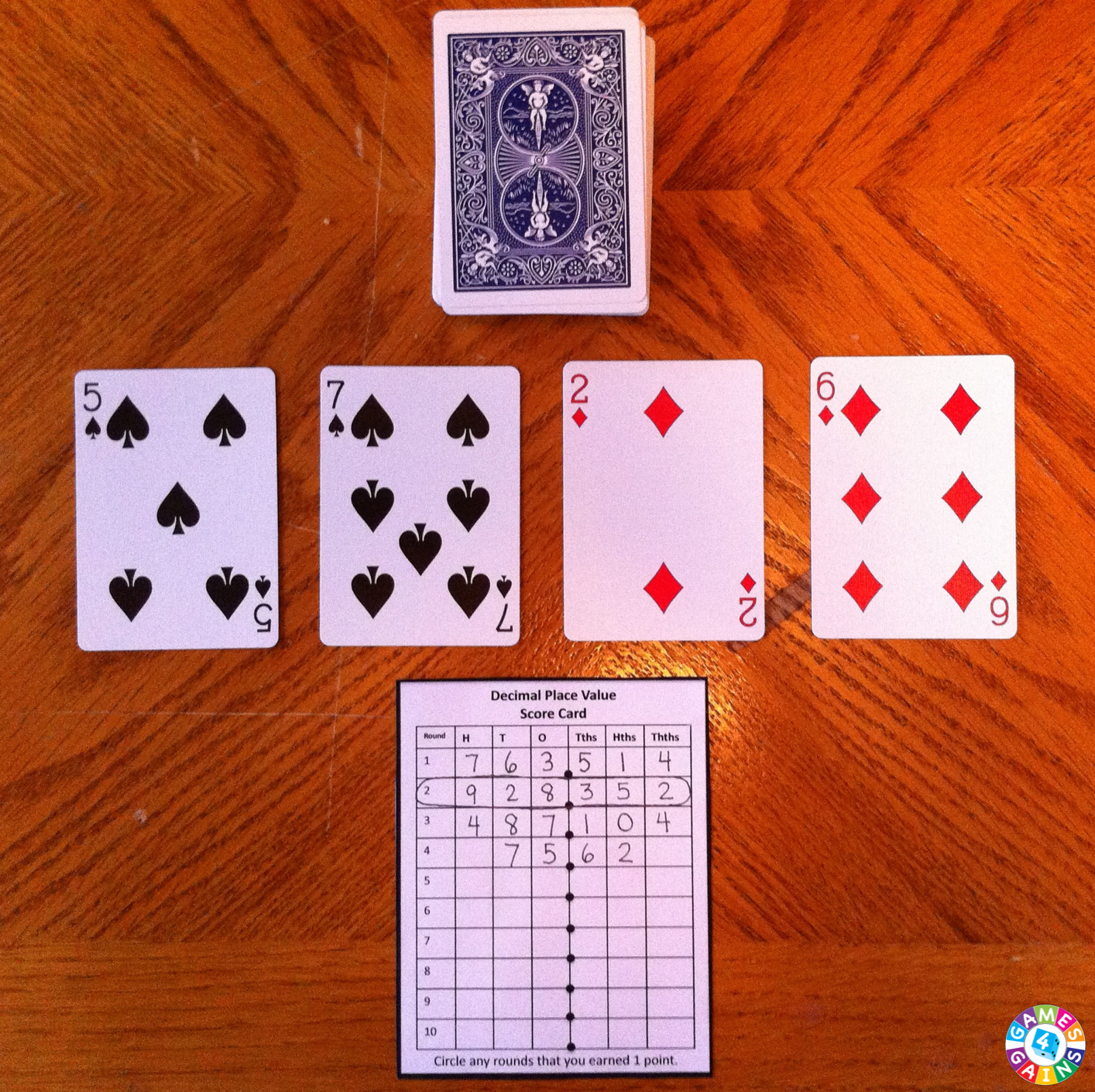7. Connect tenths and hundredths to dimes and pennies. Questions like, which is larger, 1.8 or 1.09? may suddenly seem easier if translated into money terms, \$1.80 or \$1.09.
8. Connect decimal numbers meterstick, since it is already divided into hundredths.

Resources:

#### 4 comments:

1.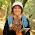Great ideas. Interestingly, my 4th grader (doing 5th grade math) is reviewing her decimals now.

2.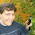Great games! I've always found the best success with the money comparison.

3.I"m going to have to read this when I'm more awake, we'll be covering decimals later this year in math I'm pretty sure.

4.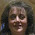Great information and resources!!! :-)

Thank you so much for taking the time to comment. It means so much.